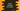# How to intersect two arrays in Kotlin# How to intersect two array elements in Kotlin :

The intersection is used to find out the common elements in two arrays. For example, if array A holds 1,2,3,4,5 and array B holds 2,5,6, the intersection between A and B will be 2,5. In this tutorial, we will learn how to find out the intersection between two array elements.

## intersect function :

Arrays come with one inbuilt function called intersect that we can use to find out the intersection between one array and one iterable. This function can be defined as below :

``````infix fun  Array.intersect(
other: Iterable
): Set``````

As you can see that this method takes one iterable as a parameter and returns one set holding common elements of the array and the iterable. The set preserves the order of the elements as the original array.

## Example :

Let’s try to understand how intersect works with an example :

``````import java.util.*

fun main(args: Array) {
//1
val firstArray = arrayOf(1,2,3,4,5)
val secondArray = arrayOf(2,5,6,7)

//2
println(Arrays.toString(firstArray))
println(Arrays.toString(secondArray))

//3
val iArray = firstArray.intersect(secondArray.toList()).toIntArray()

//4
println(Arrays.toString(iArray))
}``````

This program will print the below output :

``````[1, 2, 3, 4, 5]
[2, 5, 6, 7]
[2, 5]``````

### Explanation :

_The commented numbers in the above program denote the steps below : _

1. First of all, create two arrays of integers firstArray and secondArray.
2. Print both arrays to the user.
3. Now, find out the intersection of these two arrays. As the return value is a set, we need to convert it to an array using toIntArray(). Also, this method takes only one iterable as a parameter. So, we are converting the array to a list while passing it to intersect.
4. The final result is stored in iArray. Print the values of the array using Arrays.toString().

## Conclusion :

In this tutorial, we have learned how to use intersect function with an array to find out the intersection between two arrays in Kotlin. We can also use intersect with other iterables like a list, set, map etc. We will publish one new post with examples for other iterables. Try to run the example shown above and drop one comment below.

You might also like :